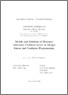# Models and Solutions of Resource Allocation Problems based on Integer Linear and Nonlinear Programming

Thomopulos, Dimitri (2016) Models and Solutions of Resource Allocation Problems based on Integer Linear and Nonlinear Programming, [Dissertation thesis], Alma Mater Studiorum Università di Bologna. Dottorato di ricerca in Automatica e ricerca operativa, 28 Ciclo. DOI 10.6092/unibo/amsdottorato/7399.
Documenti full-text disponibili:Anteprima
Documento PDF (English) - Richiede un lettore di PDF come Xpdf o Adobe Acrobat Reader

## Abstract

In this thesis we deal with two problems of resource allocation solved through a Mixed-Integer Linear Programming approach and a Mixed-Integer Nonlinear Chance Constraint Programming approach. In the first part we propose a framework to model general guillotine restrictions in two dimensional cutting problems formulated as Mixed-Integer Linear Programs (MILP). The modeling framework requires a pseudo-polynomial number of variables and constraints, which can be effectively enumerated for medium-size instances. Our modeling of general guillotine cuts is the first one that, once it is implemented within a state of-the-art MIP solver, can tackle instances of challenging size. Our objective is to propose a way of modeling general guillotine cuts via Mixed Integer Linear Programs (MILP), i.e., we do not limit the number of stages (restriction (ii)), nor impose the cuts to be restricted (restriction (iii)). We only ask the cuts to be guillotine ones (restriction (i)). We mainly concentrate our analysis on the Guillotine Two Dimensional Knapsack Problem (G2KP), for which a model, and an exact procedure able to significantly improve the computational performance, are given. In the second part we present a Branch-and-Cut algorithm for a class of Nonlinear Chance Constrained Mathematical Optimization Problems with a finite number of scenarios. This class corresponds to the problems that can be reformulated as Deterministic Convex Mixed-Integer Nonlinear Programming problems, but the size of the reformulation is large and quickly becomes impractical as the number of scenarios grows. We apply the Branch-and-Cut algorithm to the Mid-Term Hydro Scheduling Problem, for which we propose a chance-constrained formulation. A computational study using data from ten hydro plants in Greece shows that the proposed methodology solves instances orders of magnitude faster than applying a general-purpose solver for Convex Mixed-Integer Nonlinear Problems to the deterministic reformulation, and scales much better with the number of scenarios.

Abstract
Tipologia del documento
Tesi di dottorato
Autore
Thomopulos, Dimitri
Supervisore
Co-supervisore
Dottorato di ricerca
Scuola di dottorato
Scienze e ingegneria dell'informazione
Ciclo
28
Coordinatore
Settore disciplinare
Settore concorsuale
Parole chiave
Two-dimensional Cutting Problems Guillotine Knapsack Problems Chance Constrained Programming Exact Algorithms Integer Linear Programming Nonlinear Programming Pricing Stochastic Programming Computational Experiments
URN:NBN
DOI
10.6092/unibo/amsdottorato/7399
Data di discussione
27 Maggio 2016
URI